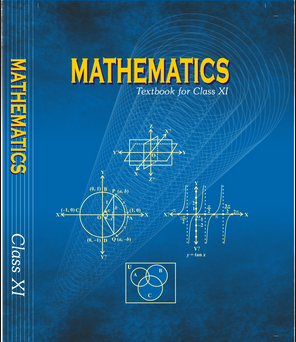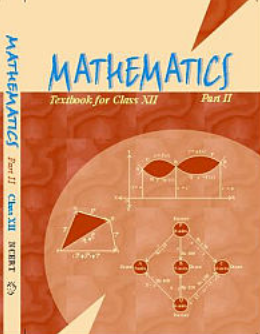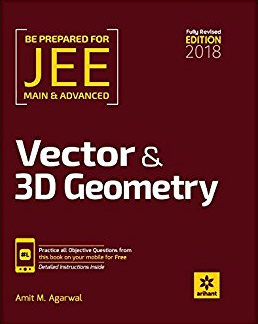# Three Dimensional Geometry (Weightage 6%)   Share

### Topics from Three Dimensional Geometry

• Coordinates of a point in space, distance between two points (10 concepts)
• Sections formula, direction rations and directio cosines, angles between two intersecting lines (13 concepts)
• Skew lines, the shortest distance between them and its equation (9 concepts)
• Equations of a line and plane in different forms, intersection of a line and a plane, coplaner lines (41 concepts)
• Rectangular Co-ordinate System in Space (2 concepts)
• Line (5 concepts)
• Plane (10 concepts)
• Sphere (1 concepts)
• Line and Plane (3 concepts)

### Important Books for Three Dimensional Geometry

•••Exams
Articles
Questions